以下是本文目录大纲：

若有不正之处请多多谅解，并欢迎批评指正。

请尊重作者劳动成果，转载请标明原文链接：

这句话从字面上看起来很容易理解，但是真正理解并不是那么容易。

我们还是先来看一个例子：

?
 1 2 3 4 5 6 7 8 9 10 11 12 13 14 15 16 `class` `ConnectionManager {` `    `  `    ``private` `static` `Connection connect = ` `null``;` `    `  `    ``public` `static` `Connection openConnection() {` `        ``if``(connect == ``null` `){` `            ``connect = DriverManager.getConnection();` `        ``}` `        ``return` `connect;` `    ``}` `    `  `    ``public` `static` `void` `closeConnection() {` `        ``if``(connect!=``null` `)` `            ``connect.close();` `    ``}` `}`

假设有这样一个数据库链接管理类，这段代码在单线程中使用是没有任何问题的，但是如果在多线程中使用呢？很显然，在多线程中使用会存在线程安全问题：第一，这里面的2个方法都没有进行同步，很可能在openConnection方法中会多次创建connect；第二，由于connect是共享变量，那么必然在调用connect的地方需要使用到同步来保障线程安全，因为很可能一个线程在使用connect进行数据库操作，而另外一个线程调用closeConnection关闭链接。

所以出于线程安全的考虑，必须将这段代码的两个方法进行同步处理，并且在调用connect的地方需要进行同步处理。

这样将会大大影响程序执行效率，因为一个线程在使用connect进行数据库操作的时候，其他线程只有等待。

那么大家来仔细分析一下这个问题，这地方到底需不需要将connect变量进行共享？事实上，是不需要的。假如每个线程中都有一个connect变量，各个线程之间对connect变量的访问实际上是没有依赖关系的，即一个线程不需要关心其他线程是否对这个connect进行了修改的。

到这里，可能会有朋友想到，既然不需要在线程之间共享这个变量，可以直接这样处理，在每个需要使用数据库连接的方法中具体使用时才创建数据库链接，然后在方法调用完毕再释放这个连接。比如下面这样：

?
 1 2 3 4 5 6 7 8 9 10 11 12 13 14 15 16 17 18 19 20 21 22 23 24 25 26 27 28 `class` `ConnectionManager {` `    `  `    ``private`  `Connection connect = ``null` `;` `    `  `    ``public` `Connection openConnection() {` `        ``if``(connect == ``null` `){` `            ``connect = DriverManager.getConnection();` `        ``}` `        ``return` `connect;` `    ``}` `    `  `    ``public` `void` `closeConnection() {` `        ``if``(connect!=``null` `)` `            ``connect.close();` `    ``}` `}`     `class` `Dao{` `    ``public` `void` `insert() {` `        ``ConnectionManager connectionManager = ``new` `ConnectionManager();` `        ``Connection connection = connectionManager.openConnection();` `        `  `        ``//使用connection进行操作` `        `  `        ``connectionManager.closeConnection();` `    ``}` `}`

这样处理确实也没有任何问题，由于每次都是在方法内部创建的连接，那么线程之间自然不存在线程安全问题。但是这样会有一个致命的影响：导致服务器压力非常大，并且严重影响程序执行性能。由于在方法中需要频繁地开启和关闭数据库连接，这样不尽严重影响程序执行效率，还可能导致服务器压力巨大。

?
 1 2 3 4 `public` `T get() { }` `public` `void` `set(T value) { }` `public` `void` `remove() { }` `protected` `T initialValue() { }`

先看下get方法的实现：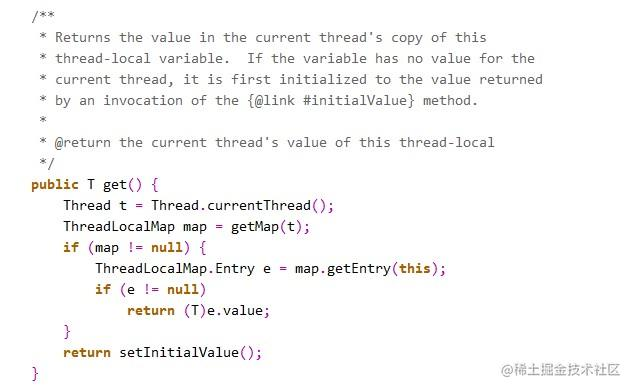如果获取成功，则返回value值。

如果map为空，则调用setInitialValue方法返回value。

我们上面的每一句来仔细分析：

首先看一下getMap方法中做了什么：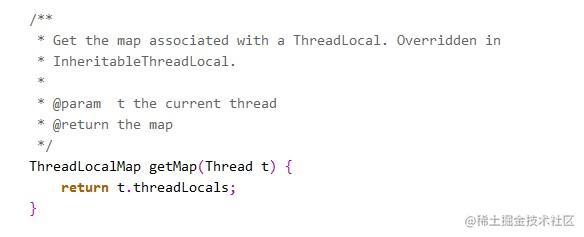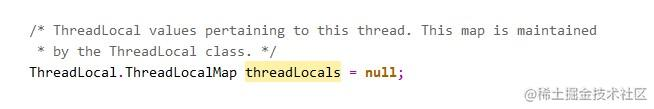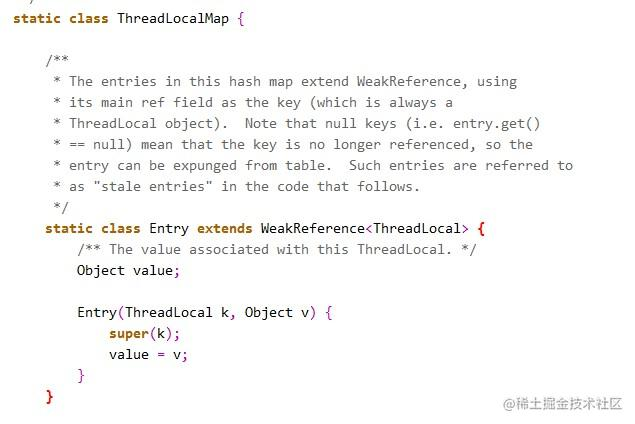然后再继续看setInitialValue方法的具体实现：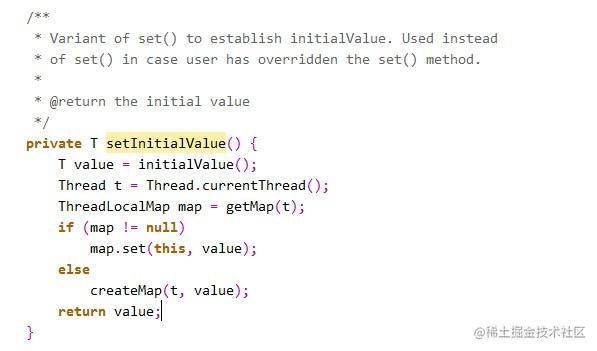很容易了解，就是如果map不为空，就设置键值对，为空，再创建Map，看一下createMap的实现：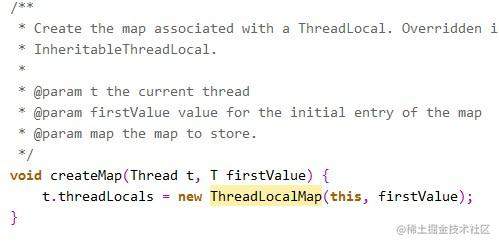?
 1 2 3 4 5 6 7 8 9 10 11 12 13 14 15 16 17 18 19 20 21 22 23 24 25 26 27 28 29 30 31 32 33 34 35 36 37 38 39 40 41 `public` `class` `Test {` `    ``ThreadLocal longLocal = ``new` `ThreadLocal();` `    ``ThreadLocal stringLocal = ``new` `ThreadLocal();`   `    `  `    ``public` `void` `set() {` `        ``longLocal.set(Thread.currentThread().getId());` `        ``stringLocal.set(Thread.currentThread().getName());` `    ``}` `    `  `    ``public` `long` `getLong() {` `        ``return` `longLocal.get();` `    ``}` `    `  `    ``public` `String getString() {` `        ``return` `stringLocal.get();` `    ``}` `    `  `    ``public` `static` `void` `main(String[] args) ` `throws` `InterruptedException {` `        ``final` `Test test = ``new` `Test();` `        `  `        `  `        ``test.set();` `        ``System.out.println(test.getLong());` `        ``System.out.println(test.getString());` `    `  `        `  `        ``Thread thread1 = ``new` `Thread(){` `            ``public` `void` `run() {` `                ``test.set();` `                ``System.out.println(test.getLong());` `                ``System.out.println(test.getString());` `            ``};` `        ``};` `        ``thread1.start();` `        ``thread1.join();` `        `  `        ``System.out.println(test.getLong());` `        ``System.out.println(test.getString());` `    ``}` `}`

这段代码的输出结果为：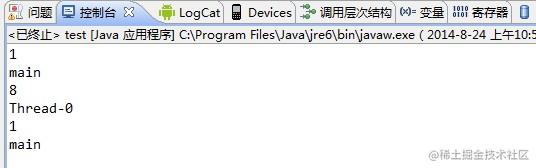总结一下：

3）在进行get之前，必须先set，否则会报空指针异常；

如果想在get之前不需要调用set就能正常访问的话，必须重写initialValue()方法。

因为在上面的代码分析过程中，我们发现如果没有先set的话，即在map中查找不到对应的存储，则会通过调用setInitialValue方法返回i，而在setInitialValue方法中，有一个语句是T value = initialValue()， 而默认情况下，initialValue方法返回的是null。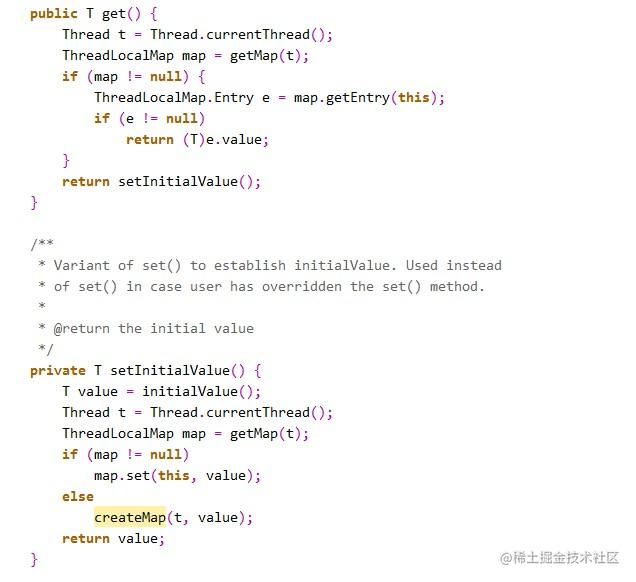看下面这个例子：

?
 1 2 3 4 5 6 7 8 9 10 11 12 13 14 15 16 17 18 19 20 21 22 23 24 25 26 27 28 29 30 31 32 33 34 35 36 37 `public` `class` `Test {` `    ``ThreadLocal longLocal = ``new` `ThreadLocal();` `    ``ThreadLocal stringLocal = ``new` `ThreadLocal();`   `    ``public` `void` `set() {` `        ``longLocal.set(Thread.currentThread().getId());` `        ``stringLocal.set(Thread.currentThread().getName());` `    ``}` `    `  `    ``public` `long` `getLong() {` `        ``return` `longLocal.get();` `    ``}` `    `  `    ``public` `String getString() {` `        ``return` `stringLocal.get();` `    ``}` `    `  `    ``public` `static` `void` `main(String[] args) ` `throws` `InterruptedException {` `        ``final` `Test test = ``new` `Test();` `        `  `        ``System.out.println(test.getLong());` `        ``System.out.println(test.getString());`   `        ``Thread thread1 = ``new` `Thread(){` `            ``public` `void` `run() {` `                ``test.set();` `                ``System.out.println(test.getLong());` `                ``System.out.println(test.getString());` `            ``};` `        ``};` `        ``thread1.start();` `        ``thread1.join();` `        `  `        ``System.out.println(test.getLong());` `        ``System.out.println(test.getString());` `    ``}` `}`

在main线程中，没有先set，直接get的话，运行时会报空指针异常。

但是如果改成下面这段代码，即重写了initialValue方法：

?
 1 2 3 4 5 6 7 8 9 10 11 12 13 14 15 16 17 18 19 20 21 22 23 24 25 26 27 28 29 30 31 32 33 34 35 36 37 38 39 40 41 42 43 44 45 46 47 48 `public` `class` `Test {` `    ``ThreadLocal longLocal = ``new` `ThreadLocal(){` `        ``protected` `Long initialValue() {` `            ``return` `Thread.currentThread().getId();` `        ``};` `    ``};` `    ``ThreadLocal stringLocal = ``new` `ThreadLocal(){;` `        ``protected` `String initialValue() {` `            ``return` `Thread.currentThread().getName();` `        ``};` `    ``};`   `    `  `    ``public` `void` `set() {` `        ``longLocal.set(Thread.currentThread().getId());` `        ``stringLocal.set(Thread.currentThread().getName());` `    ``}` `    `  `    ``public` `long` `getLong() {` `        ``return` `longLocal.get();` `    ``}` `    `  `    ``public` `String getString() {` `        ``return` `stringLocal.get();` `    ``}` `    `  `    ``public` `static` `void` `main(String[] args) ``throws` `InterruptedException {` `        ``final` `Test test = ``new` `Test();`   `        ``test.set();` `        ``System.out.println(test.getLong());` `        ``System.out.println(test.getString());` `    `  `        `  `        ``Thread thread1 = ``new` `Thread(){` `            ``public` `void` `run() {` `                ``test.set();` `                ``System.out.println(test.getLong());` `                ``System.out.println(test.getString());` `            ``};` `        ``};` `        ``thread1.start();` `        ``thread1.join();` `        `  `        ``System.out.println(test.getLong());` `        ``System.out.println(test.getString());` `    ``}` `}`

就可以直接不用先set而直接调用get了。

如：

?
 1 2 3 4 5 6 7 8 9 10 `private` `static` `ThreadLocal connectionHolder` `= ``new` `ThreadLocal() {` `public` `Connection initialValue() {` `    ``return` `DriverManager.getConnection(DB_URL);` `}` `};`   `public` `static` `Connection getConnection() {` `return` `connectionHolder.get();` `}`

下面这段代码摘自：

?
 1 2 3 4 5 6 7 8 9 10 11 12 13 14 `private` `static` `final` `ThreadLocal threadSession = ``new` `ThreadLocal();`   `public` `static` `Session getSession() ``throws` `InfrastructureException {` `    ``Session s = (Session) threadSession.get();` `    ``try` `{` `        ``if` `(s == ``null``) {` `            ``s = getSessionFactory().openSession();` `            ``threadSession.set(s);` `        ``}` `    ``} ``catch` `(HibernateException ex) {` `        ``throw` `new` `InfrastructureException(ex);` `    ``}` `    ``return` `s;` `}`

参考资料：

《深入理解Java虚拟机》

《Java编程思想》